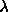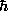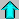# Astronomy 12 - Spring 1999 (S.T. Myers)

## Extra Credit Problem Set A (due Thu 11 Feb 1999 5pm)

Last update: Thursday, February 4, 1999

This problem set is meant as a sample for the first Midterm Exam, covering the material that was not on the first two homework sets. You may also complete it by the due date, and hand it in for extra credit. Note that you should also review the problems from the previous homeworks, as well as the reading assignments and/or other review materials that will be posted on the course page.

Problems:
1. A star of class A0 on the main seqence (Type A0 V) has a typical luminosity of 54 L_sun, and an effective temperature of 9520 K. What is the radius of this star in R_sun?

A giant star of the same class A0 (Type A0 III) has a luminosity of 106 L_sun, and a temperature of 10100 K. What is the radius of this star in R_sun?

An A0 supergiant (Type A0 Iab) has a luminosity of 35000 L_sun and temperature 9730 K. What is its radius in R_sun?

Why are all these stars considered to be A0 stars, despite their differences? Where to they lie relative to each other on the HR diagram?

2. An O8 main sequence star has a luminosity of 170000 L_sun and a mass of 23 M_sun. If the Sun has a lifetime of 10 billion years on the main sequence, approximately how long will the O8 star last?

An M8 dwarf star, probably the wimpiest main sequence star known, has a luminosity of only 0.0012 \$L_\odot\$ and a mass of 0.06 \$M_\odot\$. What would its lifetime be?

If the O8 star has a radius of 11 R_sun, and the M8 star a radius of 0.17 R_sun, estimate their effective temperatures (in K).

3. A particular star is found to have an effective blackbody temperature of 2800 K, and a luminosity of 1.2 x 10^32 Watts. What is the approximate radius of this star? How does this compare to the dimensions of objects or orbits in our solar system?

Using the H-R diagrams in Chapter 13 of Zeilik to determine the spectral type (OBAFGKML) and MK luminosity (I--VI) class of this star.

4. In lecture, we found that He II, singly ionized helium that has a single electron and a nucleus with charge +2e and is thus hydrogen-like, has energy levels that are four times those of H I, with a ground state energy of -E_0 = -54.4 eV, and level spacings of

En = -E0 n-2.

The hydrogen Balmer series consists of transitions from the n=2 levels to the higher levels n >2, and has wavelengths

1 /mn = ( 1 / 911.8 Å ) [ 1/m2 - 1/n2 ].

There is a corresponding Balmer series for singly-ionized He II. Find the formula for the wavelengths of the He II Balmer series where m=2 and n>2. Use it to find the wavelengths of He II Alpha (m=2,n=3), Beta (m=2,n=4), and the Balmer limit (m=2,n=infinity), in Ångstroms. What part of the electromagnetic spectrum are these in?

5. A large number of species of molecules have been detected in interstellar molecular clouds. One of the most important is CO (carbon monoxide) which has strong lines in the millimeter-wave band. The line due to the transition from the ground state to the first rotational (angular momentum) excited state with an energy difference of 4.768 x 10^-4 electron volts (eV). What is the frequency (in Hz) and wavelength (mm) of the line corresponding to this transition? If this line is indeed due to the J = 0 --> 1 transition, and

E = (2/2I ) J (J+1)

is the energy of angular momentum state J, what will be the frequency and wavelength of the J = 1 --> 2 transition? What is the moment of inertia I (kg m^2) of the CO molecule?Astro12 Index ---Astro12 Home

smyers@nrao.edu Steven T. Myers